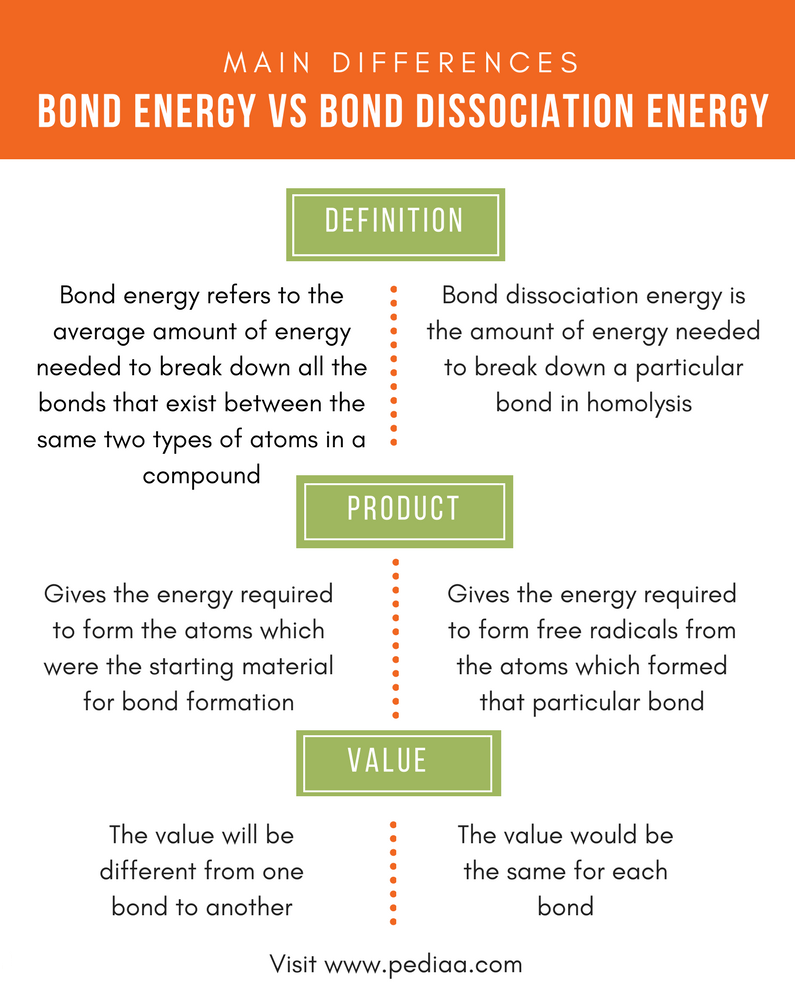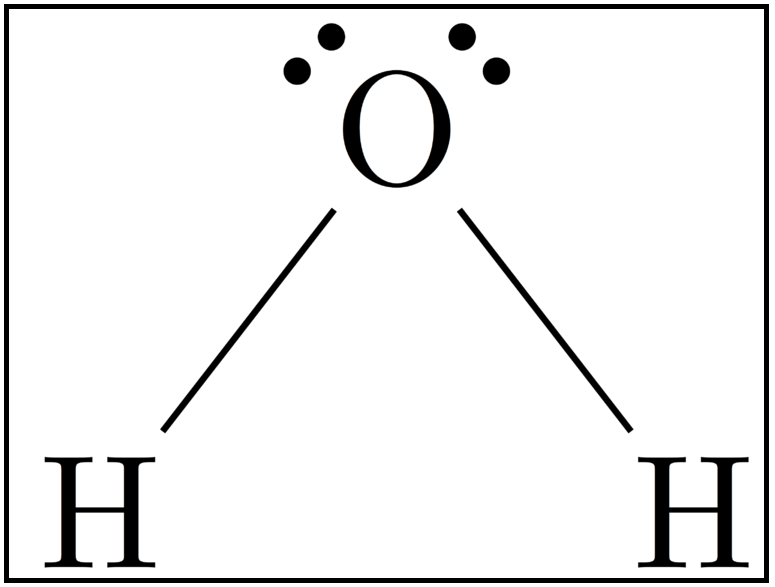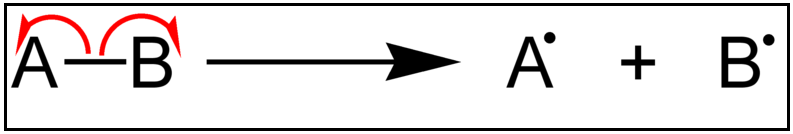# Difference Between Bond Energy and Bond Dissociation Energy

## Main Difference – Bond Energy vs Bond Dissociation Energy

The bond energy and bond dissociation energy are terms that often seem confusing since both are related to the breakdown of a chemical bond between two atoms. The concepts of bond energy and bond dissociation energy are typically used in relation to covalent bonds. This is because covalent bonds, unlike ionic bonds, are direct linkages between atoms formed due to sharing of electrons. The main difference between bond energy and bond dissociation energy is that bond energy refers to the average amount of energy needed to break down all bonds between the same two types of atoms in a compound whereas bond dissociation energy is the amount of energy needed to break down a particular bond in homolysis. In other words, bond energy is the average of bond dissociation energies of all bonds existing between the same types of atoms.

### Key Areas Covered

1. What is Bond Energy
– Definition, Unit of Calculation, Examples
2. What is Bond Dissociation Energy
– Definition, Examples
3. What is the difference between Bond Energy and Bond Dissociation Energy
– Comparison of Key Differences

Key Terms: Bond Energy, Dissociation Energy, Chemical Bond, Atoms, Homolysis, Free Radicles## What is Bond Energy

Bond energy is defined as the average amount of energy needed to break down all bonds that exist between same two types of atoms in a compound. Normally, atoms bind with each other in order to reduce their energy and obtain a lower energy level. By doing this, atoms become stable. When the bonding occurs, a certain amount of energy is released. This energy is often released as heat. Therefore, some amount of energy should be given in order to break the bond.

The unit which bond energy calculated is kjmol-1. The bond energy is different for molecules having one, two or three bonds between the same atoms. For example, C-C single bond requires 347 kJmol-1 bond energy to break that bond whereas C=C double bond requires 614 kJmol-1. But the amount of energy required for C=C is not the double value of that of C-C single bond. Hence, the bond energies are different from one bond type to another.

The best example that can be taken into account when considering the difference between bond energy and bond dissociation energy is the water (H2O) molecule.Figure: The structure of H2O

The H2O molecule is composed of two O-H bonds bonded as H-O-H. The bond energy for H2O is the average of energies required to break the two O-H bonds of the H2O molecule. Although the two bonds are the same, the energy values slightly differ because the first O-H bond is broken from H-OH structure whereas the second bond is broken as .O-H. Since an oxygen atom is more electronegative than a hydrogen atom, breaking O-H bond from water molecule is influenced by the presence or absence of hydrogen atoms on both sides of oxygen atom. Therefore, the average is taken as the bond energy.

## What is Bond Dissociation Energy

Bond dissociation energy can be defined as the amount of energy needed to break down a particular bond in homolysis. It measures the strength of a chemical bond. Bond dissociation energy is also related to covalent bonds. Since a covalent bond is formed when electrons are shared between atoms, theses shared electrons are taken back by the atoms in bond dissociation process. Hence, the radicals formed are very reactive since they have unpaired electrons. Thus, a homolysis cleavage occurs.

If the same example H2O is taken here, the bond dissociation of H2O molecule will form .OH radical and .H radical by homolysis cleavage as shown below.

H2O      +      energy     →    .OH      +     .H

Therefore, what is actually given by bond dissociation energy is the energy required to break one bond of –O-H; thus, the same value is given for both –O-H bonds.Figure 2: Homolysis of a Chemical Bond

## Difference Between Bond Energy and Bond Dissociation Energy

### Definition

Bond Energy: Bond energy refers to the average amount of energy needed to break down all the bonds that exist between the same two types of atoms in a compound.

Bond Dissociation Energy: Bond dissociation energy is the amount of energy needed to break down a particular bond in homolysis.

### Product

Bond Energy: Bond energy gives the energy required to form the atoms which were the starting material for bond formation.

Bond Dissociation Energy: Bond dissociation energy gives the energy required to form free radicals from the atoms which formed that particular bond.

### Value for Energy

Bond Energy: The value will be different from one bond to another.

Bond Dissociation Energy: The value would be the same for each bond.

### Conclusion

Both bond energy and bond dissociation energy are important in calculating the energy required for formation or breakdown of a particular compound. Although bond energy and bond dissociation energy seem the same by overview, they are two distinct forms. The main difference between bond energy and bond dissociation energy is that bond energy is the average amount of energy needed to break down all the bonds between the same two types of atoms in a compound whereas bond dissociation energy is the amount of energy needed to break down a particular bond in homolysis. In other words, bond energy is the average of bond dissociation energies of all bonds that exist between same types of atoms.

##### References:

1.” Bond Dissociation Energies of Organic Molecules.” Chemistry LibreTexts. Libretexts, 21 July 2016. Web. Available here. 13 June 2017.

##### Image Courtesy:

1. “H2O Lewis Structure PNG” By Daviewales – Own work (CC BY-SA 4.0) via Commons Wikimedia
2. “Homolysis (Chemistry)” By Jürgen Martens (Public Domain) via Commons Wikimedia